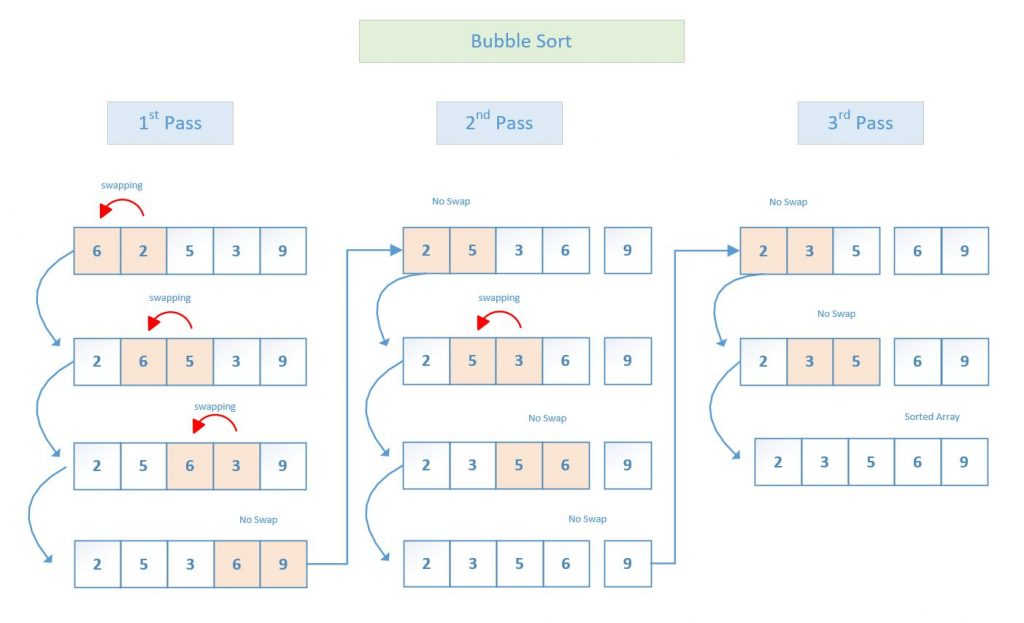# Bubble Sort

Sorting algorithm is probably one of the first thing we learn when we study computer science.  My goal is to try to provide the simplest tutorial for you that hopefully will inspire you to think in simple way, and learn the underlying data structure and algorithm.

What is bubble sort?  It is basically the idea that you continue to take 2 items at a time, compare and put them in the right order.  Here is an example illustration:There can be many implementation of this concept, so I like to share a  pretty simple and optimized bubble sort algorithm in Java.  Here is the code:

``` public static void bubble_sort(int numArray[]) {

boolean swapped = true;
int last = numArray.length - 2;

while (swapped) {
swapped = false; //if we go through this loop and never swap, the array is sorted

for (int i=0; i<= last; i++) {
if (numArray[i] > numArray[i+1]) {
int temp = numArray[i];
numArray[i] = numArray[i+1];
numArray[i+1] = temp;
swapped = true; //we did a swap, so the array is not sorted yet
}
}
last--;
}
}```

Here is what I like to highlight:

• as we keep comparing and moving the largest number toward the end of the array, the end of the array get sorted first. So we can actually stop comparing them, and our working set is getting smaller and smaller.
• we need to pay attention to the case when the numbers are sorted, so that we don’t waste time comparing the sorted array over and over again.  We do that by keeping track if we did any swap in the last pass.  If we did a swap, that mean the numbers were not sorted yet, we we did not do a swap, that mean that the numbers are already sorted.

With these optimizations, it helps to reduce the sorting time for some data.  And if you sort large amount of data, you will definitely be able to see the benefit in performance pretty clearly.

If you are interested, here is a link to my Github repository with the full source code.  It also has some added diagnostic print outs to show some statistics to you. Oh, by the way, it also has an overloaded method to accept an array of strings, so that you can also sort text in addition to numbers.

Happy coding !